# Matching Supply with Demand

## Quiz 11 :Betting on Uncertain Demand: the Newsvendor ModelLooking for Management Homework Help?

## Quiz 11 :Betting on Uncertain Demand: the Newsvendor Model(McClure Books ) Dan McClure owns a thriving independent bookstore in artsy New Hope, Pennsylvania. He must decide how many copies to order of a new book, Power and Self-Destruction , an exposé on a famous politician's lurid affairs. Interest in the book will be intense at first and then fizzle quickly as attention turns to other celebrities. The book's retail price is $20 and the wholesale price is$12. The publisher will buy back the retailer's leftover copies at a full refund, but McClure Books incurs $4 in shipping and handling costs for each book returned to the publisher. Dan believes his demand forecast can be represented by a normal distribution with mean 200 and standard deviation 80. a. Dan will consider this book to be a blockbuster for him if it sells more than 400 units. What is the probability Power and Self-Destruction will be a blockbuster b. Dan considers a book a "dog" if it sells less than 50 percent of his mean forecast. What is the probability this exposé is a "dog" c. What is the probability demand for this book will be within 20 percent of the mean forecast d. What order quantity maximizes Dan's expected profit e. Dan prides himself on good customer service. In fact, his motto is "McClure's got what you want to read." How many books should Dan order if he wants to achieve a 95 percent in-stock probability f. If Dan orders the quantity chosen in part e to achieve a 95 percent in-stock probability, then what is the probability that "Dan won't have what some customer wants to read" (i.e., what is the probability some customer won't be able to purchase a copy of the book) g. Suppose Dan orders 300 copies of the book. What would Dan's expected profit be in this case Free Essay Answer: Answer: In a single-period inventory model, the order size decision is made by a marginal analysis. In this analysis, the optimal order quantity occurs at the point where the marginal benefit of stocking one additional unit is just less than its expected marginal cost. In a single-period inventory model, increase the order size as long as the probability of selling what is ordered in less than or equal to the critical ratio ( CR ) defined as: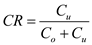Where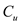and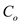are the cost per unit of demand underestimated and that of demand overestimated respectively. a. Compute the probability of having the demand, D ~ N (200, 80) more than or equal to 400 units.* Note: In MS-Excel,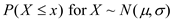is computed as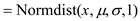. So, the probability of having more than 400 units of demand is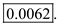b. Compute the probability of having the demand, D ~ N (200, 80) less than or equal to 50% of the mean forecast.So, the probability of the book becoming a "dog" is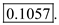c. Compute the probability of having the demand, D ~ N (200, 80) within 20% of the mean forecast.So, the required probability is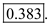d.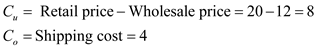Compute the critical ratio using the following formula: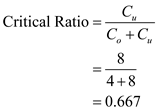For optimal order quantity, Q , the distribution function, F(Q) must be equal to the critical ratio. F(Q) = 0.667. Compute the standard normal variable z as follows: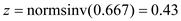Compute corresponding order quantity using the following method.Since a fractional order quantity is infeasible, the optimal order quantity is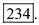e. Compute the order quantity given the in-stock probability as follows: The in-stock probability or F(Q) = 0.95 Compute the standard normal variable z as follows: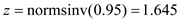Compute corresponding order quantity using the following method.Since a fractional order quantity is infeasible, the order quantity is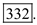f. If the in-stock probability is 95%, the stock-out probability must be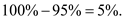So, the probability that some customers won't be able to purchase a copy is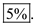g. Given the order size, compute the expected profit using the following method. Order size, Q = 300For z = 1.25, the corresponding loss function from the Exhibit 12.4 is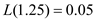Expected lost sales,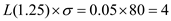So,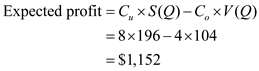So, the expected profit is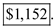( EcoTable Tea ) EcoTable is a retailer of specialty organic and ecologically friendly foods. In one of their Cambridge, Massachusetts, stores, they plan to offer a gift basket of Tanzanian teas for the holiday season. They plan on placing one order and any leftover inventory will be discounted at the end of the season. Expected demand for this store is 4.5 units and demand should be Poisson distributed. The gift basket sells for$55, the purchase cost to EcoTable is $32, and leftover baskets will be sold for$20. a. If they purchase only 3 baskets, what is the probability that some demand will not be satisfied b. If they purchase 10 baskets, what is the probability that they will have to mark down at least 3 baskets c. How many baskets should EcoTable purchase to maximize its expected profit d. Suppose they purchase 4 baskets. How many baskets can they expect to sell e. Suppose they purchase 6 baskets. How many baskets should they expect to have to mark down at the end of the season f. Suppose EcoTable wants to minimize its inventory while satisfying all demand with at least a 90 percent probability. How many baskets should they order g. Suppose EcoTable orders 8 baskets. What is its expected profit
Free
Essay

In a single-period inventory model, the order size decision is made by a marginal analysis. In this analysis, the optimal order quantity occurs at the point where the marginal benefit of stocking one additional unit is just less than its expected marginal cost.
In a single-period inventory model, increase the order size as long as the probability of selling what is ordered in less than or equal to the critical ratio ( CR ) defined as:Whereandare the cost per unit of demand underestimated and that of demand overestimated respectively.
a.
Use the following method to find the probability of some demand not being satisfied.
Note that Demand, D ~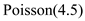The stock-out happens when the demand is more that the order quantity which is 3 units.So, the stock-out probability or the probability of some demand not being satisfied is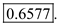b.
The mark down requirement in 3 or more when the demand is 7 or less given the purchase quantity is 10.So, the probability of marking down 3 or more units is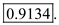c.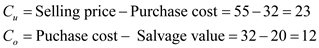Compute the critical ratio using the following formula: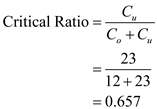For optimal order quantity, Q , the distribution function, F(Q) must be equal to the critical ratio. F(Q) = 0.657.
From the Poisson distribution table, F(4) = 0.5321 and F(5) = 0.7029. Since F(5) 0.657, select order quantity of 5 units
Therefore, the required order quantity is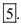d.
For four baskets, using the Poisson loss function table, the loss function value is 1.088.
Therefore, the expected sales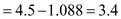So, the expected sold units are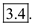e.
For six baskets, using the Poisson loss function table, the loss function value is 0.323.
Therefore, the expected sales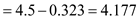So, the left-over units for mark-down are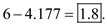f.
Given the in-stock probability requirement of 90%, find the required purchase quantity using the following method:
Using the Poisson distribution table, F(6) = 0.831 and F(7) = 0.913. Since F(7) 0.90, select order quantity of 7 units.
Therefore, the required order quantity is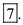g.
Given the order size, compute the expected profit using the following method.
For eight baskets, using the Poisson loss function table, the loss function value is 0.0676.
Therefore, the expected sales,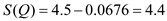So, the left-over units for mark-down are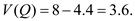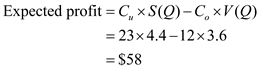So, the expected profit is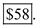(Pony Express Creations) Pony Express Creations Inc. (www.pony-ex.com) is a manufacturer of party hats, primarily for the Halloween season. (80 percent of their yearly sales occur over a six-week period.) One of their popular products is the Elvis wig, complete with sideburns and metallic glasses. The Elvis wig is produced in China, so Pony Express must make a single order well in advance of the upcoming season. Ryan, the owner of Pony Express, expects demand to be 25,000 and the following is his entire demand forecast: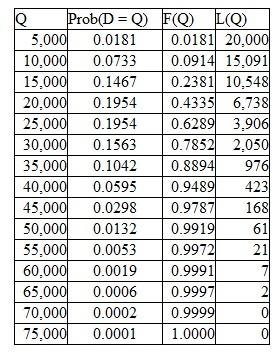Prob( D = Q ) = Probability demand D equals Q F ( Q ) = Probability demand is Q or lower L ( Q ) = Expected lost sales if Q units are ordered The Elvis wig retails for $25, but Pony Express's wholesale price is$12. Their production cost is $6. Leftover inventory can be sold to discounters for$2.50. a. Suppose Pony Express orders 40,000 Elvis wigs. What is the chance they have to liquidate 10,000 or more wigs with a discounter b. What order quantity maximizes Pony Express's expected profit c. If Pony Express wants to have a 90 percent in-stock probability, then how many Elvis wigs should be ordered d. If Pony Express orders 50,000 units, then how many wigs can they expect to have to liquidate with discounters e. If Pony Express insists on a 100 percent in-stock probability for its customers, then what is its expected profit
Free
Essay

In a single-period inventory model, the order size decision is made by a marginal analysis. In this analysis, the optimal order quantity occurs at the point where the marginal benefit of stocking one additional unit is just less than its expected marginal cost.
In a single-period inventory model, increase the order size as long as the probability of selling what is ordered in less than or equal to the critical ratio ( CR ) defined as:Whereandare the cost per unit of demand underestimated and that of demand overestimated respectively.
a.
The liquidation requirement in 10,000 or more when the demand is 30,000 or less given the production quantity is 40,000.
For Q = 30,000, F(Q) = 0.7852
So, the probability of having 1,000 units or more being liquidated is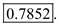b.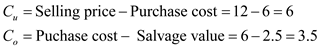Compute the critical ratio using the following formula: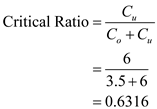For optimal order quantity, Q , the distribution function, F(Q) must be equal to the critical ratio. F(Q) = 0.6316.
From the empirical distribution table, for Q = 30,000, F(Q) = 0.7852 which is greater than the optimality requirement of F(Q).
So, the optimal production quantity is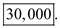c.
Given the in-stock probability requirement of 90%, find the required production quantity using the following method:
F(Q) = 0.90
From the empirical distribution table, for Q = 40,000, F(Q) = 0.9489 which is greater than the optimality requirement of F(Q).
Therefore, the required production quantity is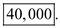d.
With the given production quantity, use the following method to compute the left-over units eligible for discount.
Production quantity, Q = 50,000
Corresponding loss function value from the empirical distribution table , L(Q) = 63
So, the Expected sales,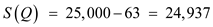So, the left-over units eligible for discount are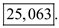e.
The 100% in-sock probability occurs when the production quantity, Q = 75,000.
Corresponding loss function value from the empirical distribution table , L(Q) = 2
So, the Expected sales,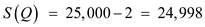So, there will be no profit. The expected loss is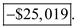(Flextrola) Flextrola, Inc., an electronics systems integrator, is planning to design a key component for their next-generation product with Solectrics. Flextrola will integrate the component with some software and then sell it to consumers. Given the short life cycles of such products and the long lead times quoted by Solectrics, Flextrola only has one opportunity to place an order with Solectrics prior to the beginning of its selling season. Flextrola's demand during the season is normally distributed with a mean of 1,000 and a standard deviation of 600. Solectrics' production cost for the component is $52 per unit and it plans to sell the component for$72 per unit to Flextrola. Flextrola incurs essentially no cost associated with the software integration and handling of each unit. Flextrola sells these units to consumers for $121 each. Flextrola can sell unsold inventory at the end of the season in a secondary electronics market for$50 each. The existing contract specifies that once Flextrola places the order, no changes are allowed to it. Also, Solectrics does not accept any returns of unsold inventory, so Flextrola must dispose of excess inventory in the secondary market. a. What is the probability that Flextrola's demand will be within 25 percent of its forecast b. What is the probability that Flextrola's demand will be more than 40 percent greater than its forecast c. Under this contract, how many units should Flextrola order to maximize its expected profit For parts d through i, assume Flextrola orders 1,200 units. d. What are Flextrola's expected sales e. How many units of inventory can Flextrola expect to sell in the secondary electronics market f. What is Flextrola's expected gross margin percentage, which is (Revenue Cost)/Revenue g. What is Flextrola's expected profit h. What is Solectrics' expected profit i. What is the probability that Flextrola has lost sales of 400 units or more j. A sharp manager at Flextrola noticed the demand forecast and became wary of assuming that demand is normally distributed. She plotted a histogram of demands from previous seasons for similar products and concluded that demand is better represented by the log normal distribution. Figure 12.7 plots the density function for both the log normal and the normal distribution, each with mean of 1,000 and standard deviation of 600. Figure 12.8 plots the distribution function for both the log normal and the normal. Using the more accurate forecast (i.e., the log normal distribution), approximately how many units should Flextrola order to maximize its expected profit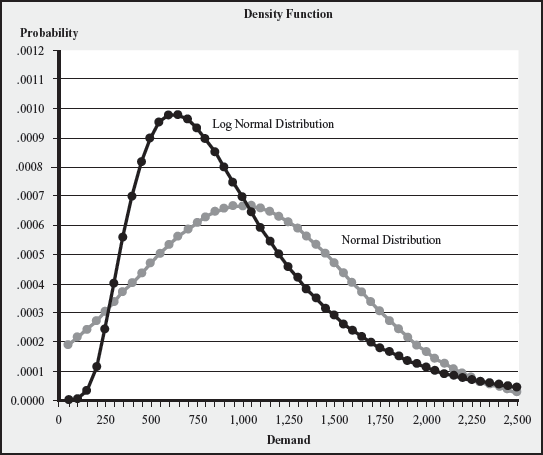FIGURE 12.7 Density Function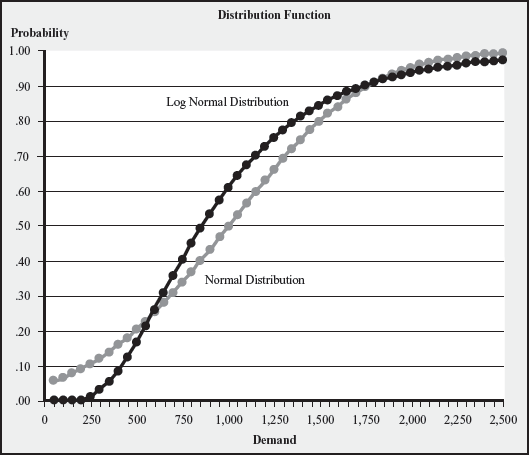FIGURE 12.8 Distribution Function
Essay(Fashionables) Fashionables is a franchisee of The Limited, the well-known retailer of fashionable clothing. Prior to the winter season, The Limited offers Fashionables the choice of five different colors of a particular sweater design. The sweaters are knit overseas by hand, and because of the lead times involved, Fashionables will need to order its assortment in advance of the selling season. As per the contracting terms offered by The Limited, Fashionables also will not be able to cancel, modify, or reorder sweaters during the selling season. Demand for each color during the season is normally distributed with a mean of 500 and a standard deviation of 200. Further, you may assume that the demands for each sweater are independent of those for a different color. The Limited offers the sweaters to Fashionables at the wholesale price of $40 per sweater and Fashionables plans to sell each sweater at the retail price of$70 per unit. The Limited delivers orders placed by Fashionables in truckloads at a cost of $2,000 per truckload. The transportation cost of$2,000 is borne by Fashionables. Assume unless otherwise specified that all the sweaters ordered by Fashionables will fit into one truckload. Also assume that all other associated costs, such as unpacking and handling, are negligible. The Limited does not accept any returns of unsold inventory. However, Fashionables can sell all of the unsold sweaters at the end of the season at the fire-sale price of $20 each. a. How many units of each sweater type should Fashionables order to maximize its expected profit b. If Fashionables wishes to ensure a 97.5 percent in-stock probability, what should its order quantity be for each type of sweater For parts c and d, assume Fashionables orders 725 of each sweater. c. What is Fashionables' expected profit d. What is the stockout probability for each sweater e. Now suppose that The Limited announces that the unit of truckload capacity is 2,500 total units of sweaters. If Fashionables orders more than 2,500 units in total (actually, from 2,501 to 5,000 units in total), it will have to pay for two truckloads. What now is Fashionables' optimal order quantity for each sweater Essay Answer:(Teddy Bower Parkas) Teddy Bower is an outdoor clothing and accessories chain that purchases a line of parkas at$10 each from its Asian supplier, TeddySports. Unfortunately, at the time of order placement, demand is still uncertain. Teddy Bower forecasts that its demand is normally distributed with mean of 2,100 and standard deviation of 1,200. Teddy Bower sells these parkas at $22 each. Unsold parkas have little salvage value; Teddy Bower simply gives them away to a charity. a. What is the probability this parka turns out to be a "dog," defined as a product that sells less than half of the forecast b. How many parkas should Teddy Bower buy from TeddySports to maximize expected profit c. If Teddy Bower wishes to ensure a 98.5 percent in-stock probability, how many parkas should it order For parts d and e, assume Teddy Bower orders 3,000 parkas. d. Evaluate Teddy Bower's expected profit. e. Evaluate Teddy Bower's stockout probability Essay Answer:(Teddy Bower Boots) To ensure a full line of outdoor clothing and accessories, the marketing department at Teddy Bower insists that they also sell waterproof hunting boots. Unfortunately, neither Teddy Bower nor TeddySports has expertise in manufacturing those kinds of boots. Therefore, Teddy Bower contacted several Taiwanese suppliers to request quotes. Due to competition, Teddy Bower knows that it cannot sell these boots for more than$54. However, $40 per boot was the best quote from the suppliers. In addition, Teddy Bower anticipates excess inventory will need to be sold off at a 50 percent discount at the end of the season. Given the$54 price, Teddy Bower's demand forecast is for 400 boots, with a standard deviation of 300. a. If Teddy Bower decides to include these boots in its assortment, how many boots should it order from its supplier b. Suppose Teddy Bower orders 380 boots. What would its expected profit be c. John Briggs, a buyer in the procurement department, overheard at lunch a discussion of the "boot problem." He suggested that Teddy Bower ask for a quantity discount from the supplier. After following up on his suggestion, the supplier responded that Teddy Bower could get a 10 percent discount if they were willing to order at least 800 boots. If the objective is to maximize expected profit, how many boots should it order given this new offer(Land's End) Geoff Gullo owns a small firm that manufactures "Gullo Sunglasses." He has the opportunity to sell a particular seasonal model to Land's End. Geoff offers Land's End two purchasing options: • Option 1. Geoff offers to set his price at $65 and agrees to credit Land's End$53 for each unit Land's End returns to Geoff at the end of the season (because those units did not sell). Since styles change each year, there is essentially no value in the returned merchandise. • Option 2. Geoff offers a price of $55 for each unit, but returns are no longer accepted. In this case, Land's End throws out unsold units at the end of the season. This season's demand for this model will be normally distributed with mean of 200 and standard deviation of 125. Land's End will sell those sunglasses for$100 each. Geoff's production cost is $25. a. How much would Land's End buy if they chose option 1 b. How much would Land's End buy if they chose optioN2 c. Which option will Land's End choose d. Suppose Land's End chooses option 1 and orders 275 units. What is Geoff Gullo's expected profit Essay Answer:(CPG Bagels) CPG Bagels starts the day with a large production run of bagels. Throughout the morning, additional bagels are produced as needed. The last bake is completed at 3 P.M. and the store closes at 8 P.M. It costs approximately$0.20 in materials and labor to make a bagel. The price of a fresh bagel is $0.60. Bagels not sold by the end of the day are sold the next day as "day old" bagels in bags of six, for$0.99 a bag. About two-thirds of the day-old bagels are sold; the remainder are just thrown away. There are many bagel flavors, but for simplicity, concentrate just on the plain bagels. The store manager predicts that demand for plain bagels from 3 P.M. until closing is normally distributed with mean of 54 and standard deviation of 21. a. How many bagels should the store have at 3 P.M. to maximize the store's expected profit (from sales between 3 P.M. until closing) ( Hint: Assume day-old bagels are sold for $0.99/6 =$0.165 each; i.e., don't worry about the fact that day-old bagels are sold in bags of six.) b. Suppose that the store manager is concerned that stockouts might cause a loss of future business. To explore this idea, the store manager feels that it is appropriate to assign a stockout cost of $5 per bagel that is demanded but not filled. (Customers frequently purchase more than one bagel at a time. This cost is per bagel demanded that is not satisfied rather than per customer that does not receive a complete order.) Given the additional stockout cost, how many bagels should the store have at 3 P.M. to maximize the store's expected profit c. Suppose the store manager has 101 bagels at 3 P.M. How many bagels should the store manager expect to have at the end of the day Essay Answer:(The Kiosk) Weekday lunch demand for spicy black bean burritos at the Kiosk, a local snack bar, is approximately Poisson with a mean of 22. The Kiosk charges$4.00 for each burrito, which are all made before the lunch crowd arrives. Virtually all burrito customers also buy a soda that is sold for 60¢. The burritos cost the Kiosk \$2.00, while sodas cost the Kiosk 5¢. Kiosk management is very sensitive about the quality of food they serve. Thus, they maintain a strict "No Old Burrito" policy, so any burrito left at the end of the day is disposed of. The distribution function of a Poisson with mean 22 is as follows: x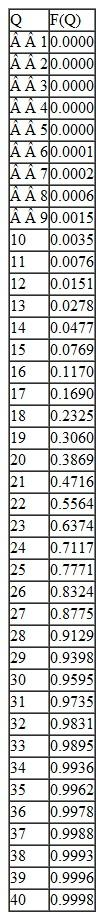a. Suppose burrito customers buy their snack somewhere else if the Kiosk is out of stock. How many burritos should the Kiosk make for the lunch crowd b. Suppose that any customer unable to purchase a burrito settles for a lunch of Pop-Tarts and a soda. Pop-Tarts sell for 75¢ and cost the Kiosk 25¢. (As Pop-Tarts and soda are easily stored, the Kiosk never runs out of these essentials.) Assuming that the Kiosk management is interested in maximizing profits, how many burritos should they prepare